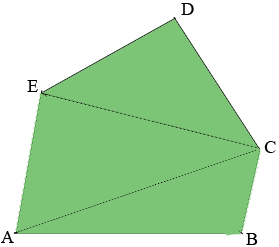SEARCH HOMEMath Central Quandaries & QueriesQuestion from Dale: I'm needing to find the square footage of an irregular pentagon. I've seen the other questions similar to this one, but without a great deal of difficulty can't get you the angle degrees, or the length between opposing angles. I'm hoping that a different bit of information can help. So here goes: The longest side is 67ft long. One of its legs is 39ft long, and the other is 18ft long. The leg off of the other end of the 39ft leg is 35ft long. The leg off of the other end of the 18ft is 49ft long, and meets the other end of the 35ft side. The information that I DO have, which I'm hoping makes the difference, is that the 39ft and the 18ft sides are parallel. Also, while the angles at each end of the 67ft side are not right angles, they are fairly close to it, approximately 80 degrees at the 39 ft side corner, and 100 degrees at the 18ft side corner. Dale.Hi Dale,

I can show you an approach to take using the angle measurements you supplied. I am going to leave much of the calculation to you.

I drew a diagram of your pentagon, not to scale, labeled the vertices and added two lines.• $|AB| = 67$ feet
• $|BC| = 18$ feet
• $|CD| = 49$ feet
• $|DE| = 35$ feet
• $|EA| = 39$ feet
• Angle $EAB$ measures $80^o$
• Angle $ABC$ measures $100^o$

Using the Law of Cosines on triangle $ABC$ I get

$|CA|^2 = |AB|^2 + |BC|^2 - 2 |AB| |BC| \cos\left(ABC\right)$

or

$|CA|^2 = 67^2 + 18^2 - 2 \times 67 \times 18 \cos(100^o) = 5231.8394 \mbox{ feet squared}$

or

$|CA| = \sqrt{5231.8394} = 72.33 \mbox{ feet.}$

At this point use the Law of Sines on triangle $ABC$ to get

$\frac{\sin(CAB)}{|BC|}=\frac{\sin(ABC)}{|AC|}$

or

$\sin(CAB) = \frac{|BC| \sin(ABC)}{|AC|} = \frac{18 \sin(100^0)}{72.33}.$

and hence

$CAB = \sin^{-1}\left(\frac{18 \sin(100^0)}{72.33}\right) = 14.19^{o}.$

Since the measure of the angle $EAB$ is $80^o$ the measure of the angle $EAC$ is $80 - 14.19 = 65.81$ degrees.

Now you can again use the Law of Cosines on the triangle $EAC$ to find the length of $CE|. At this point you have partitioned the pentagon into three triangles$ABC, CEA$and$CDE.$You know the lengths of the three sides of each of the triangles so you can use Heron's Formula to find the area of each of the triangles and the area of the pentagon is the sum of the areas of these triangles. Since you seem to be somewhat sure of the measure of the angle$ABC$you could modify it slightly, say to 97 degrees, making the measure of the angle$EAB\$ 83 degrees, repeat the calculations and see how much effect it has on the area of the pentagon.

Write back if you need more assistance.

Harley* Registered trade mark of Imperial Oil Limited. Used under license.Math Central is supported by the University of Regina and the Imperial Oil Foundation.